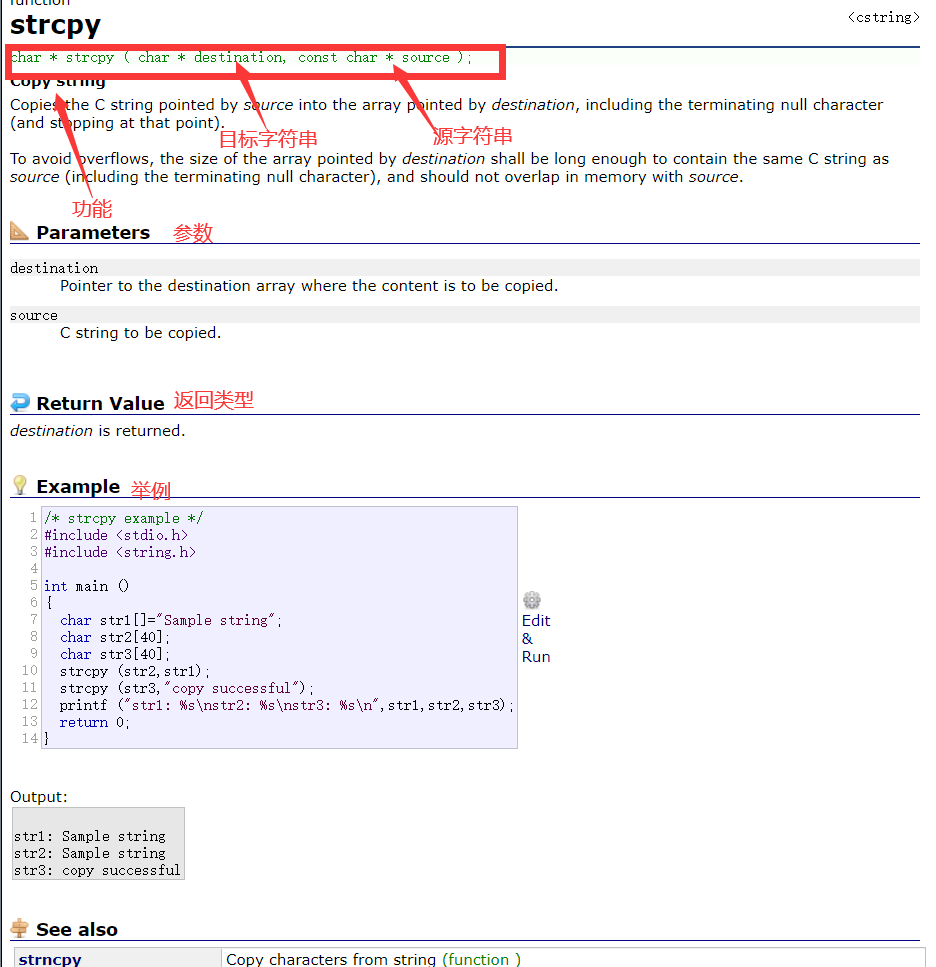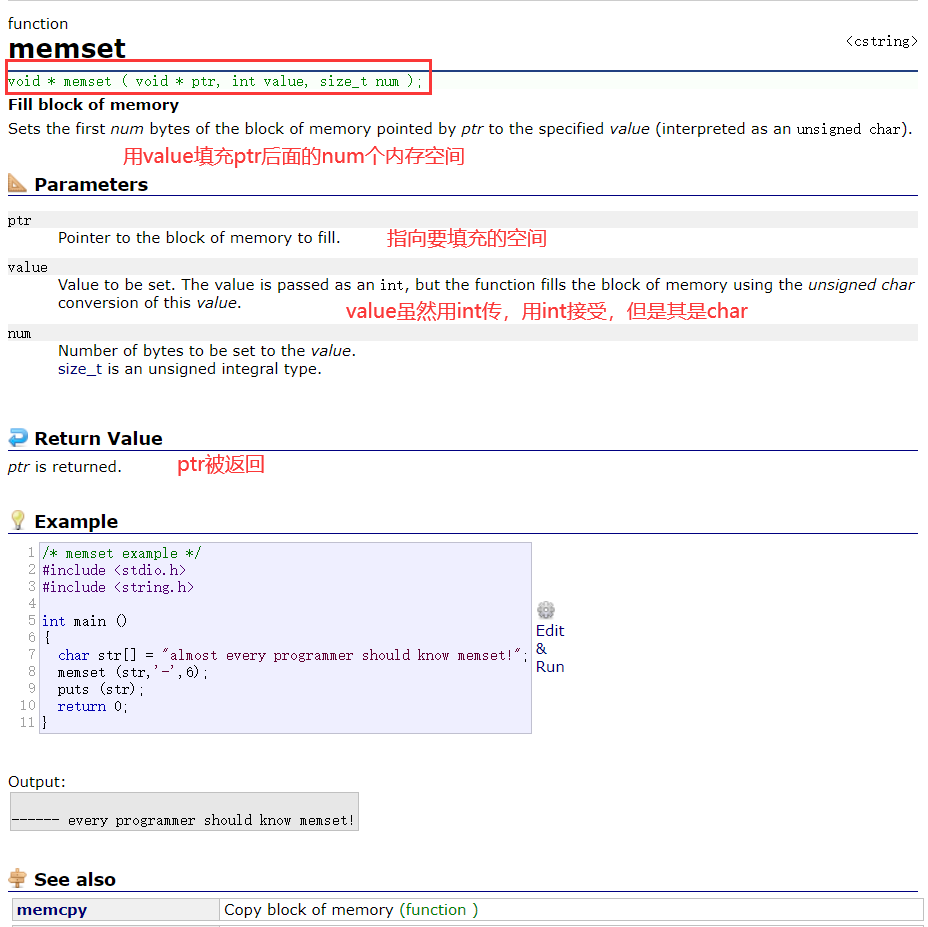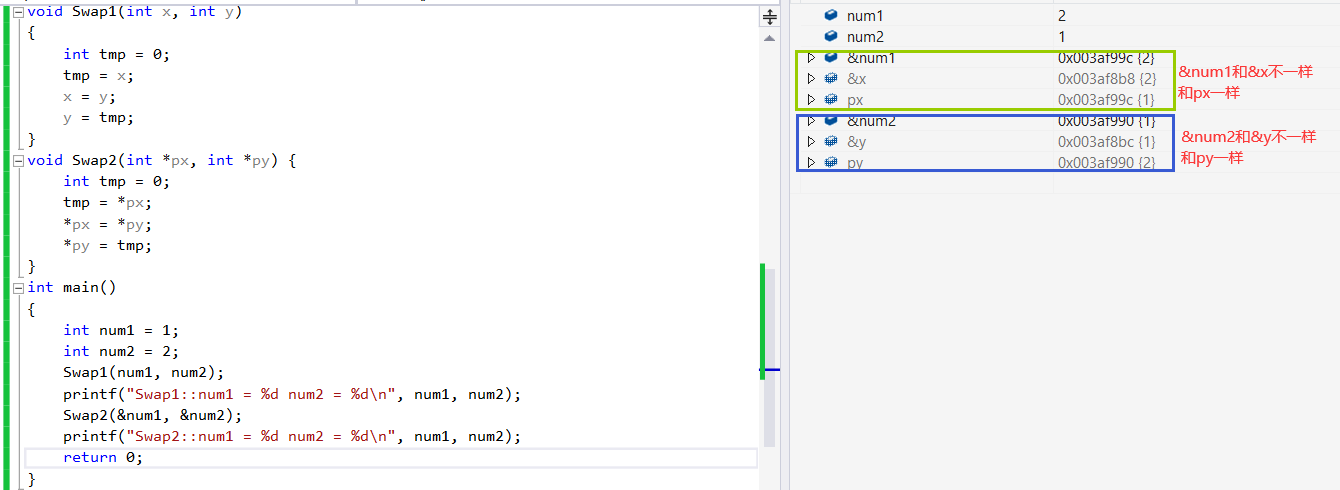WHCSRL 技术网

# 11.函数(C语言)

## 1.函数是什么？

1）函数是一个大型程序中的某部分代码， 由一个或多个语句块组成。它负责完成某项特定任务，而且相较于其他代 码，具备相对的独立性。

2）一般会有输入参数并有返回值，提供对过程的封装和细节的隐藏。这些代码通常被集成为软件库。

## 1）库函数的基本知识

1. 我们知道在我们学习C语言编程的时候，总是在一个代码编写完成之后迫不及待的想知道结果，想
把这个结果打印到我们的屏幕上看看。这个时候我们会频繁的使用一个功能：将信息按照一定的格
式打印到屏幕上（printf）。
2. 在编程的过程中我们会频繁的做一些字符串的拷贝工作（strcpy）。
3. 在编程是我们也计算，总是会计算n的k次方这样的运算（pow）。
像上面我们描述的基础功能，它们不是业务性的代码。我们在开发的过程中每个程序员都可能用的到，
为了支持可移植性和提高程序的效率，所以C语言的基础库中提供了一系列类似的库函数，方便程序员进行软件开发。

MSDN(Microsoft Developer Network)
www.cplusplus.com
http://en.cppreference.com（英文版）
http://en.cppreference.com（中文版）

## 2）初识几个库函数

1.strcpy``````//strcpy
int main()
{
char ch1[] = "hello bit";
char ch2 = { 0 };
strcpy(ch2, ch1);
printf("%%s
%%s
", ch1, ch2);
return 0;
}

```12345678910```

2.memset``````//memset
int main()
{
char ch[] = "hello bit, you are strong!";
memset(ch, 'o', 5);//将ch指向的内存后面的5个空间用‘o’填充
printf("%%s
", ch);
return 0;
}
```12345678```

## 自定义函数的组成

``````ret_type fun_name(para1, * )
{
statement;//语句项
}
ret_type 返回类型
fun_name 函数名
para1    函数参数
```1234567```

``````#include <stdio.h>
//get_max函数的设计
int get_max(int x, int y) {
return (x>y)?(x):(y);
}
int main()
{
int num1 = 10;
int num2 = 20;
int max = get_max(num1, num2);
printf("max = %%d
", max);
return 0
```123456789101112```

## 4和5示例分析：

``````//交换两个数的值
void Swap1(int x, int y)
{
int tmp = 0;
tmp = x;
x = y;
y = tmp;
}
void Swap2(int *px, int *py) {
int tmp = 0;
tmp = *px;
*px = *py;
*py = tmp;
}
int main()
{
int num1 = 1;
int num2 = 2;
Swap1(num1, num2);//传值调用
printf("Swap1::num1 = %%d num2 = %%d
", num1, num2);
Swap2(&num1, &num2);//传址调用
printf("Swap2::num1 = %%d num2 = %%d
", num1, num2);
return 0;
}

```1234567891011121314151617181920212223242526```1.上面Swap1和Swap2函数中的参数 x，y，px，py 都是形式参数.

2.Swap1是传值调用，明显没有与函数外面取得呼应
Swap2是传址调用，明显与函数外部取得联系，使num1和num2的值交换

## 1. 写一个函数可以判断一个数是不是素数

``````#include<math.h>
int Is_prime(int x)
{
int i = 0;
for (i = 2; i <= sqrt(x); i ++)
{
if (x%%i == 0)
{
return 0;
}
}
return 1;
}

int main()
{
int num = 0;
scanf("%%d", &num);
int ret = Is_prime(num);
printf("%%d
", ret);
if (ret)//0为假，其他为真
printf("是素数
");
else
printf("不是素数
");
return 0;
}
```1234567891011121314151617181920212223242526```

## 3. 写一个函数，实现一个整形有序数组的二分查找

``````int binary_search(int arr[], int k, int sz)
{
int left = 0;
int right = sz-1;
while (left <= right)
{
int mid = (left + right) / 2;
if (arr[mid] > k)
{
right = mid - 1;
}
else if (arr[mid] < k)
{
left = mid + 1;
}
else
{
return mid;
}
}
return -1;
}
int main()
{
int arr = { 1, 2, 3, 4, 5, 6, 7, 8, 9, 10 };
int k = 7;
int sz = sizeof(arr) / sizeof(arr);
int ret = binary_search(arr, k, sz);//arr是数组第一个元素的地址，k为要查找的数，len为数组的大小
if (ret == -1)
{
printf("找不到
");
}
else
{
printf("找到了，下标为%%d
", ret);
}
return 0;
}
```1234567891011121314151617181920212223242526272829303132333435363738```